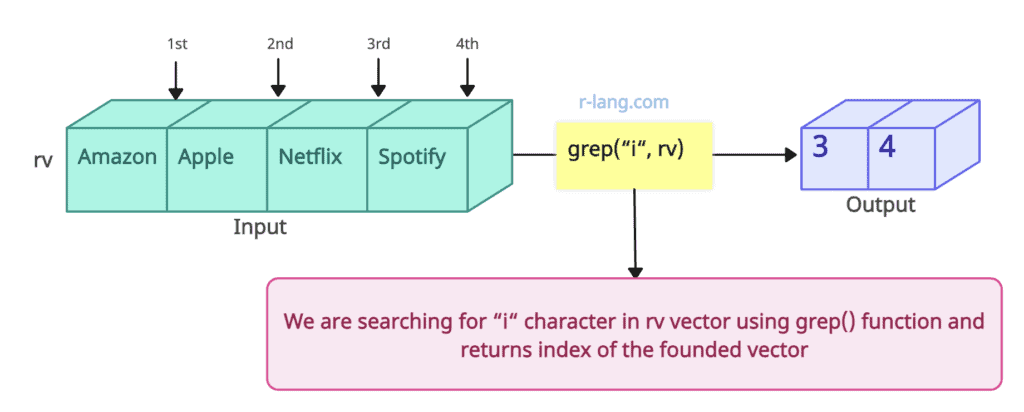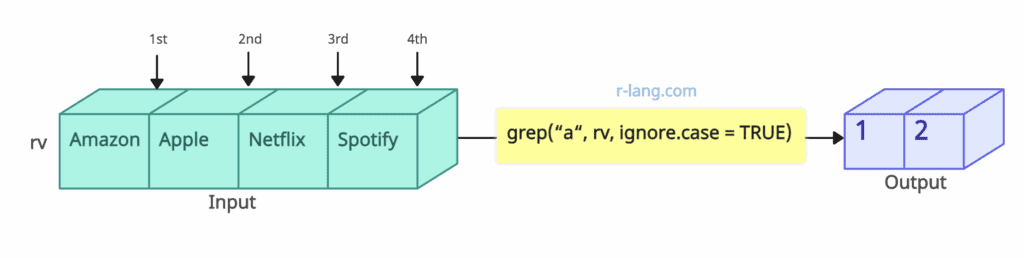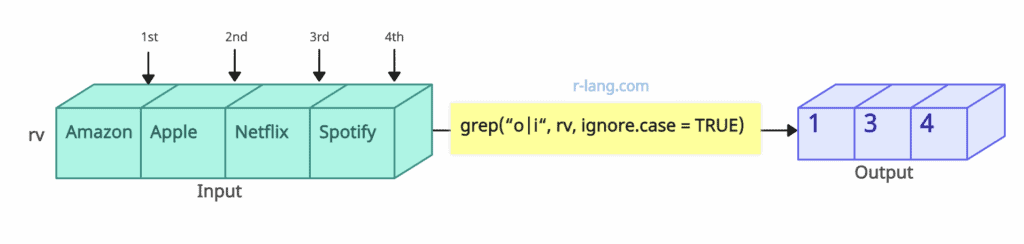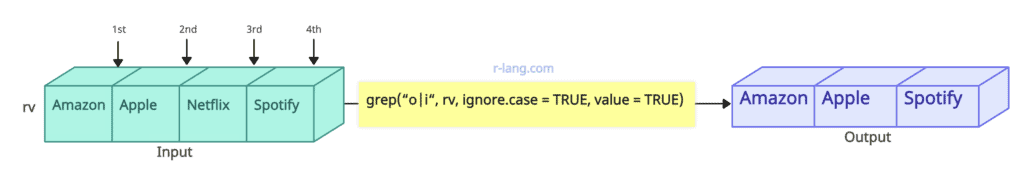# How to Use grep() Function in R

The grep() function in R is used to check for matches of characters or sequences of characters in a given string.

## Syntax

``grep(pattern, x, ignore.case = FALSE, value = FALSE)``

## Parameters

1. pattern: It takes a character string containing a regular expression (or character string for fixed = TRUE) to match the given character vector.
2. x: It is a character vector where matches are sought, or an object can be coerced by as.character to a character vector.
3. ignore.case: If FALSE, the pattern matching is case sensitive, and if TRUE, the case is ignored during matching.
4. value: If it is a FALSE, a vector containing the (integer) indices of the matches determined by grep() is returned, and if TRUE, a vector containing the matching elements is returned.

## Return Value

It returns a vector of the indices of the elements of x that yielded a match.

## Example 1: How to Use grep() function

Let’s search for the character “i” in the character string.``````rv <- c("Amazon", "Apple", "Netflix", "Spotify")

print(grep("i", rv))``````

Output

`` 3  4``

## Example 2: Passing ignore.case argumentIn the above figure, we are searching for the character “a” in our input character vector. The “a” character does not exist in the input character vector, but “A” exists. Because we are passing “ignore.case = TRUE”, that means now, it will look for “a” or “A” and since “A” exists, it will return the index for the vector that contains A.

``````rv <- c("Amazon", "Apple", "Netflix", "Spotify")

print(grep("a", rv, ignore.case = TRUE))
``````

Output

`````` 1  2
``````

## Example 3: Passing multiple patterns

The grep() function can check for multiple character patterns in the vector of character strings and returns the indices of elements that contain the pattern.``````rv <- c("Amazon", "Apple", "Netflix", "Spotify")

print(grep("o|i", rv, ignore.case = TRUE))``````

Output

`` 1  3  4``

In this code, the grep() function searches for elements containing either “o” or “i” and returns their indices.

## Example 4: Passing the “value = TRUE” argument

If you set the argument value=TRUE, it will return the actual matched elements themselves instead of their indices.``````rv <- c("Amazon", "Apple", "Netflix", "Spotify")

print(grep("o|i", rv, ignore.case = TRUE, value = TRUE))
``````

Output

`````` "Amazon" "Netflix" "Spotify"
``````

That’s it.